## Calculate the price of a bond with an odd last coupon before the last coupon period

Oct 18

Written by: Charles Flock
10/18/2011 8:09 PM11 Financial Calculations that you can’t do in EXCEL - Part 10 of 11
10. Calculate the price of a bond with an odd last coupon before the last coupon period.
Enter the following information in a worksheet.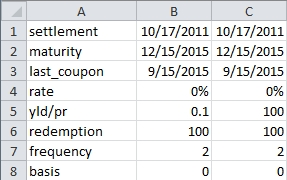Enter this formula in cell B9: =ODDLPRICE(B1,B2,B3,B4,B5,B6,B7,B8). Enter this formula in C9: =ODDLYIELD(C1,C2,C3,C4,C5,C6,C7,C8). You should get the following result.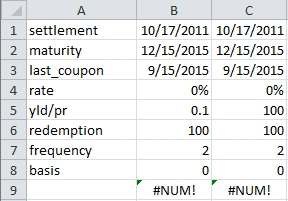Now, go and change the settlement value to 10/17/2015. You should get the following result.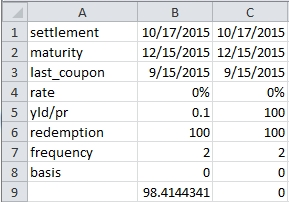What happened there? One answer is that the software is working according to the documentation. The documentation actually has the following statement:
The following date condition must be satisfied; otherwise, ODDYIELD returns the #NUM! error value:
Maturity > settlement > last_interest
Really? That’s the rule? So, what does EXCEL mean by last_interest? According to the EXCEL documentation, last_interest is the security’s last coupon date. For most of the rest of the world, this would be last coupon date before the maturity date. Is it possible, that EXCEL has a different definition, perhaps it means the latest coupon date before the settlement date? Of course, under that definition, that means that the last_interest would be changing over the life of the bond and you would need to recalculate it. And, what would that recalculation be based on? Why, the last coupon date before the maturity date!
This is more than a terminology problem, however. Bonds with odd last coupon periods can either have a short last period (meaning that the coupon will be less than the normal periodic coupon) or a long last period (meaning that the coupon will be more than the normal periodic coupon). If the function is really requiring that the last coupon date to be the latest coupon date before the settlement date, there is no way for the function to distinguish between odd-long and odd-short last periods.
In our example above, where we have specified on odd-short last period, it would be quite acceptable (at least from a computation point of view), for the last coupon date to have been the 15th of March rather that the 15th of September. If the last coupon date in the function must be less than the settlement date, there is absolutely no way for the function to correctly identify the last coupon date before the maturity date.
Having said all of that, is it possible to get any meaningful price information from this function? Let’s revert to our original settlement date and change the last_interest to be the latest coupon date before the settlement date. This is what our spreadsheet should look like: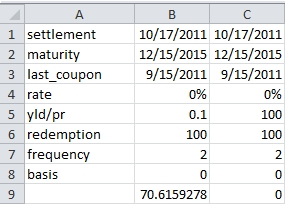That looks like a more reasonable answer. Is it right? How can we go about checking it?
Since this is a zero-coupon bond, there is only one cash flow at maturity, so we can use the LOG function to determine what value the EXCEL ODDLPRICE function used as the exponent for determining the price.
Enter the following formula in CELL B10: =LOG(B6/B9)/LOG(1+B5/B7); and this formula in B11: =POWER(1+B5/B7,-B10)*B6. Your worksheet should now look like this: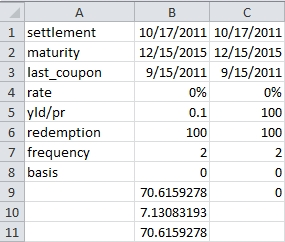We can see that exponent value in B10 will return the value calculated by ODDLPRICE in B9. Now we can just verify that value. Since the basis value is 0 and the frequency is 2, there are 180 days in each period. So, we just need to calculate the number of days from the settlement date to next coupon date (we can us the COUPDAYSNC function to do that) divided by 180, the number of coupons from next coupon date until the last coupon date, and the number of days from the last coupon date to the quasi maturity date divide by 180 (using the COUPDAYBS function). You should end up with a sheet that looks something like this.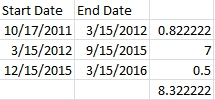So this seems to be saying that the exponent should be 8.32222 and not 7.13083193. It seems that ODDLPRICE function is off by more than a full coupon period.
Can we use that PRICE function to help us out here? Since the maturity period is exactly one-half of a coupon and since this is a zero-coupon bond, the PRICE function and the ODDLPRICE function should return the same value. Enter the following formula in B12: =PRICE(B1,B2,B4,B5,B6,B7,B8) and this formula in B13: =LOG(B6/B12)/LOG(1+B5/B7). Your worksheet should now look like this.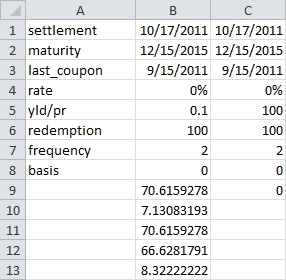Look at B13. This is exactly the value that we predicted. It looks like we cannot use the ODDLPRICE function in EXCEL.
Here’s the result in SQL Server.
SELECT wct.ODDLPRICE(
'10/17/2011'     --settlement
,'12/15/2015'     --maturity
,'09/15/2015'     --last interest
,.00              --rate
,.10              --yld
,100              --redemption
,2                --frequency
,1                --basis
)
This produces the following result.
----------------------
66.6218286232342

(1 row(s) affected)

This, of course, is the value that EXCEL should have produced.

 Previous: Calculate the interest payment amount on a pay in advance loan
 Next: Calculate a PMT amount when there is an odd first period

Tags:
Categories:
Location: BlogsThe WestClinTech Blog

## Search Blogs

 KeywordsPhrase

## Blog Archives

Archive
 < June 2023 >
SunMonTueWedThuFriSat
28293031123
45678910
11121314151617
18192021222324
2526272829301
2345678
Monthly
Go

### SupportCopyright 2008-2023 Westclintech LLC         Privacy Policy        Terms of Service